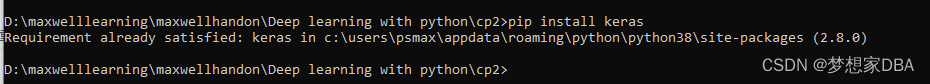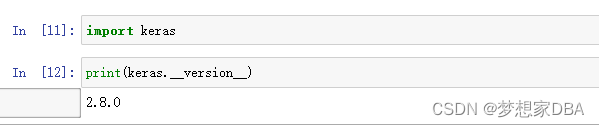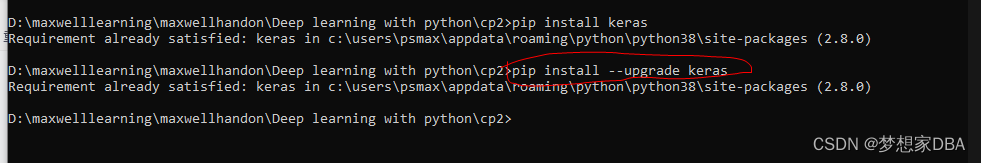﻿• 价格透明
• 信息保密
• 进度掌控
• 售后无忧# Introduction to Keras

Two of the top numerical platforms in Python that provide the basic for deep learning research and development are Theano and TensorFlow. Both are very powerful libraries, but both can be difficult to use directly for creating deep learning models. In this lesson you will discover the Keras Python library that provides a clean and convenient way to create a range of deep learning modules on top of Theano or TensorFlow. After completiing this lesson you will know:

• About the Keras Python library for deep learning
• How to configure Keras for Theano or TensorFlow.
• The standard idiom for creating models with Keras.

## 4.1 What is Keras?

Keras is a minimalist Python library for deep learning that can run on top of Theano or TensorFlow. It was developed to make developing deep learning models as fast and easy as possible for research and development.It is released under the permissive MIT license. Keras was developed and maintained by Fran¸cois Chollet, a Google engineer using four guiding principles:

• Modularity:A model can be understood as a sequence or a graph alone.
• Minimalism: The library provides just enough to achieve an outcome, no frills and maximizing readability.
• Extensibility:New components are intentionally easy to add and use within the framework, intended for developers to trial and explore new ideas.
• Python:No separate model files with custom file formats. Everything is native Python.

## 4.2 How to Install Keras

Keras can be installed easily using pip:

``sudo pip install keras````sudo pip install --upgrade keras``## 4.3 Theano and TensorFlow Backends for Keras

Keras is a lightweight API and rather than providing an implementation of the required mathematical operations needed for deep learning it provides a consistent interface to efficient numerical libraries called backends.

## 4.4 Build Deep Learning Models with Keras

The focus of Keras is the idea of a model. The main type of model is a sequence of layers called a Sequential which is a linear stack of layers. You create a Sequential and add layers to it in the order that you wish for the computation to be performed. Once defined, you compile the model which makes use of the underlying framework to optimize the computation to be performed by your model. In this you can specify the loss function and the optimizer to be used.We can summarize the construction of deep learning models in Keras as follows:

1. Define your model.  Create a Sequential model and add configured layers.
2. Compile your model. Specify loss function and optimizers and call the compile() function on the model.
3. Fit your model. Train the model on a sample of data by calling the fit() function on the model.
4. Make predictions. Use the model to generate predictions on new data by calling functions such as evaluate() or predict() on the model.

## 4.5 Summary

In this lesson you discovered the Keras Python library for deep learning research and development. You learned:

• Keras wraps both the TensorFlow and Theano libraries, abstracting their capabilities and hiding their complexity.
• Keras is designed for minimalism and modularity allowing you to very quickly define deep learning models.
• Keras deep learning models can be developed using an idiom of defining, compiling and fitting models that can then be evaluated or used to make predictions.

### 4.5.1 Next

You are now up to speed with the Python libraries for deep learning. In the next project you will discover step-by-step how you can develop and run very large deep learning models using these libraries in the cloud using GPU hardware at a fraction of the cost of purchasing your own hardware.### 低价透明### 金牌服务### 信息保密